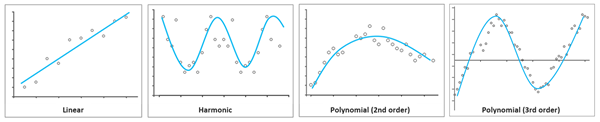# 生成趋势栅格 (Image Analyst)

## 使用方法

• 此工具的一个使用示例是：您有 40 年的月度海洋温度数据，且您希望为每个像素拟合趋势线，以查看温度随时间变化的位置和方式。

• 支持的多维栅格数据集包括 netCDF、GRIB、HDF 和 Esri 的 CRF。此外，还支持多维镶嵌数据集。

• 此工具可生成采用云栅格格式 (CRF) 的多维栅格数据集。目前尚不支持其他输出格式。

• 默认情况下，将使用 LZ77 压缩类型压缩多维栅格输出。但是，建议您将压缩类型更改为 LERC，并根据您的数据调整最大误差。例如，如果您希望将分析结果精确到三位小数，则请将最大误差设置为 0.001。最好避免不必要的精度要求，因为这会导致处理时间和存储大小增加。

• 系统提供三个趋势线选项用于沿维度拟合变量值的趋势：线性、谐波和多项式。三个趋势拟合选项如下所述。• 线性 - 线性趋势线是最佳拟合直线，可用于估计简单的线性关系。线性趋势可突出显示以稳定速率增加或减少的变化率。线性趋势线的公式如下：
• y = 像素变量值
• x = 维度值
• ß0 = y 截距
• ß1 = 线性斜率或变化率

ß1 > 0 表示增加趋势

ß1 < 0 表示减少趋势

• 谐波 - 谐波趋势线是周期性重复的曲线，最适用于描述遵循周期性模式的数据，例如季节性温度变化。谐波趋势线的公式如下：
• y = 像素变量值
• t = 儒略日
• ß0 = y 截距
• ß1 = 变化率
• α, γ = 年际或年内变化的系数
• ω = i
• f = 谐波频率
• 多项式 - 多项式趋势线是一条曲线，可用于波动的数据。在这种情况下，多项式的阶数值用于指示发生的最大波动数。多项式趋势线的公式如下：
• y = 像素变量值
• x = 维度值
• ß0, ß1, ß2, ß3, ..., ßn = 常系数
• 使用此工具生成的输出趋势栅格可用作使用趋势栅格预测工具的输入。输出是一个多维栅格，其中每个剖切均是一个包含趋势线相关信息的多波段栅格。如果要分析包含单个维度（例如，时间）的数据集的单个变量趋势，则输出数据集中将具有单个剖切。如果要分析包含多个维度（例如，时间和深度）的数据集的单个变量，则每个剖切将包含沿分析中未包含维度的每个维度值的趋势信息。

对于线性趋势分析，输出包含三波段栅格，其中：

• 波段 1 = 斜率
• 波段 2 = 截距
• 波段 3 = 均方根误差 (RMSE) 或最佳拟合线周围的误差

对于谐波趋势分析，输出中的波段数取决于谐波频率。当频率设置为 1 时，输出将为五波段栅格，其中：

• 波段 1 = 斜率
• 波段 2 = 截距
• 波段 3 = Harmonic_sin1
• 波段 4 = Harmonic_cos1
• 波段 5 = RMSE

当频率设置为 2 时，输出将为七波段栅格，其中：

• 波段 1 = 斜率
• 波段 2 = 截距
• 波段 3 = Harmonic_sin1
• 波段 4 = Harmonic_cos1
• 波段 5 = Harmonic_sin2
• 波段 6 = Harmonic_cos2
• 波段 7 = RMSE

对于多项式趋势分析，输出中的波段数取决于多项式阶数。二阶多项式拟合将生成四波段栅格，其中：

• 波段 1 = Polynomial_2
• 波段 2 = Polynomial_1
• 波段 3 = Polynomial_0
• 波段 4 = RMSE

三阶多项式拟合将生成五波段栅格，其中：

• 波段 1 = Polynomial_3
• 波段 2 = Polynomial_2
• 波段 3 = Polynomial_1
• 波段 4 = Polynomial_0
• 波段 5 = RMSE

• 谐波趋势分析的周期长度参数用于表示您希望在一天或全年的数据中看到的周期数量和周期长度。例如，如果您希望数据在一年中经历两个变化周期，则周期长度将为 182.5 天或 0.5 年。如果您每三小时采集一次温度数据，并且每天存在一个变化周期，则周期长度将为 1 天。

• 谐波趋势分析的频率参数用于描述谐波模型以拟合至数据。如果频率设置为 1，则将使用线性和一阶谐波曲线的组合来拟合模型。如果频率为 2，则将使用线性、一阶谐波和二阶谐波曲线的组合来拟合数据。如果频率为 3，则将使用附加的三阶谐波曲线对数据进行建模，依此类推。

• 可以生成模型拟合优度统计数据作为可选输出。可以计算均方根误差 (RMSE)、R 平方和趋势斜率 p 值并在输出栅格的属性窗口的统计数据部分中显示。也可以通过使用 RGB 符号系统符号化输出趋势栅格并将统计数据指定为红色、绿色和蓝色波段来显示统计数据。

## 语法

`GenerateTrendRaster(in_multidimensional_raster, dimension, {variables}, {line_type}, {frequency}, {ignore_nodata}, {cycle_length}, {cycle_unit}, {rmse}, {r2}, {slope_p_value})`
 参数 说明 数据类型 in_multidimensional_raster 输入多维栅格数据集。 Raster Dataset; Raster Layer; Mosaic Dataset; Mosaic Layer; Image Service; File dimension 将沿此维度为分析中所选的一个或多个变量提取趋势。 String variables[variables,...]（可选） 将计算趋势的一个或多个变量。如果未指定变量，则将分析多维栅格中的第一个变量。 String line_type（可选） 指定用于拟合沿维度像素值的线的类型。LINEAR —将沿线性趋势线拟合变量像素值。这是默认设置。POLYNOMIAL —将沿二阶多项式趋势线拟合变量像素值。HARMONIC —将沿谐波趋势线拟合变量像素值。 String frequency（可选） 趋势拟合中使用的频率或多项式阶数。如果趋势类型是多项式，则此参数将指定多项式阶数。如果趋势类型为谐波，则此参数将指定用于拟合趋势的模型数量。仅当分析的维度是时间时，趋势分析中才会包含此参数。如果 line_type 参数为 HARMONIC，则默认值为 1，这意味着将使用一阶谐波曲线来拟合模型。如果 line_type 参数是 POLYNOMIAL，则默认值为 2 或二阶多项式。 Long ignore_nodata（可选） 指定分析中是否忽略 NoData 值。DATA — 分析将包括沿给定维度的所有有效像素，并忽略所有 NoData 像素。这是默认设置。NODATA —如果沿给定维度的像素包含任意 NoData 值，则分析结果将变为 NoData。 Boolean cycle_length（可选） 要进行建模的周期性变化的长度。line_type 设置为 HARMONIC 时需要此参数。例如，叶绿度通常在一年中具有一个较强的变化周期，因此周期长度为 1 年。每小时温度数据在一天中具有一个较强的变化周期，因此周期长度为 1 天。对于每年周期变化的数据，默认长度为 1 年。 Double cycle_unit（可选） 指定用于谐波周期长度的时间单位。DAYS —谐波周期的长度单位为天。YEARS —谐波周期的长度单位为年。这是默认设置。 String rmse（可选） 指定是否将计算趋势拟合线的均方根误差 (RMSE)。RMSE —将计算 RMSE。这是默认设置。NO_RMSE —将不计算 RMSE。 Boolean r2（可选） 指定是否将为趋势拟合线计算 R 平方拟合优度统计数据。R2 —将计算 R 平方值。NO_R2 —将不计算 R 平方值。这是默认设置。 Boolean slope_p_value（可选） 指定是否将为趋势线的斜率系数计算 p 值统计数据。SLOPEPVALUE —将计算 p 值。NO_SLOPEPVALUE —将不计算 p 值。这是默认设置。 Boolean

#### 返回值

 名称 说明 数据类型 out_multidimensional_raster 输出云栅格格式 (CRF) 多维栅格数据集。 Raster

## 代码示例

GenerateTrendRaster 示例 1（Python 窗口）

``````# Import system modules
import arcpy
from arcpy.ia import *

# Check out the ArcGIS Image Analyst extension license
arcpy.CheckOutExtension("ImageAnalyst")

# Execute
trendCoeffMultidim = arcpy.ia.GenerateTrendRaster("model_time_series.crf",
"StdTime", "precip", "POLYNOMIAL", 2, "DATA", None, '', "RMSE","R2", None)

# Save output
trendCoeffMultidim.save("C:/data/polynomial_trend_coefficients.crf")``````
GenerateTrendRaster 示例 2（独立脚本）

``````# Import system modules
import arcpy
from arcpy.ia import *

# Check out the ArcGIS Image Analyst extension license
arcpy.CheckOutExtension("ImageAnalyst")

# Define input parameters

inFile = "C:/Data/ClimateVariables.crf"
dimensionName = "Depth"
variables = "salinity;temp"
trend_type = "LINEAR"
frequency = None
ignore_Nodata = "DATA"
cycle_length = None
cycle_unit = ''
rmse = "RMSE"
r2 = "R2"
slopeP = "NO_SLOPEPVALUE")

# Execute - fit a linear model for salinity and temperature
trendCoeffMultidim = GenerateTrendRaster(inFile, dimensionName,
variables, trend_type, frequency, ignore_nodata, cycle_length,
cycle_unit, rmse, r2, slopeP)

# Save output
trendCoeffMultidim.save("C:/data/linear_trend_coefficients.crf")``````
GenerateTrendRaster 示例 3（独立脚本）

``````# Import system modules
import arcpy
from arcpy.ia import *

# Check out the ArcGIS Image Analyst extension license
arcpy.CheckOutExtension("ImageAnalyst")

# Define input parameters

inFile = "C:/data/ndvi_time_series.crf"
dimensionName = "StdTime"
variables = "ndvi"
trend_model = "HARMONIC"
harmonic_frequency = 1
ignore_Nodata = "DATA"
length_of_cycle = 1
cycle_unit = "YEARS"
rmse = "RMSE"
r_square = "NO_R2"
p_value = "NO_SLOPEPVALUE"

# Execute
trendCoeffMultidim = GenerateTrendRaster(inFile, dimensionName,
variables, trend_model, harmonic_frequency, ignore_nodata,
length_of_cycle, cycle_unit, rmse,r_square,p_value)

# Save output
trendCoeffMultidim.save("C:/data/harmonic_trend_coefficients.crf")``````

## 许可信息

• Basic: 需要 Image Analyst
• Standard: 需要 Image Analyst